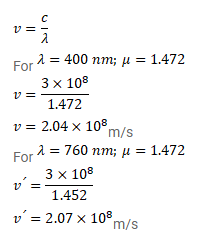# The index of refraction of fused quartz isQuestion:

The index of refraction of fused quartz is $1.472$ for light of wavelength $400 \mathrm{~nm}$ and is $1.452$ for light of wavelength $760 \mathrm{~nm}$. Find the speeds of light of these wavelengths in fused quartz.

Solution: